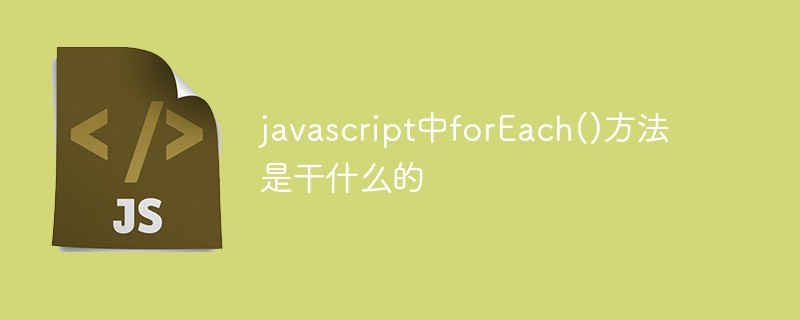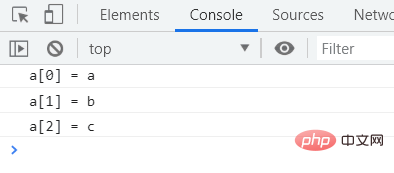# javascript中forEach()方法是干什么的Array 类型为每个数组定义了 forEach() 原型方法，使用该方法可以为数组执行迭代操作。

forEach() 方法用于调用数组的每个元素，并将元素传递给回调函数。

`array.forEach(function(currentValue, index, arr), thisValue)`

• array：一个数组对象。

• function(currentValue, index, arr)：必需参数，最多可以接收三个参数的函数。forEach 将为数组中的每个元素调用 callbackfn 函数一次。

• currentValue：必需。当前元素

• index：可选。当前元素的索引值。

• arr：可选。当前元素所属的数组对象。

• thisArg：可选参数，callbackfn 函数中的 this 可引用的对象。如果省略 thisArg，则 this 的值为 undefined。

forEach 方法不直接修改原始数组，但回调函数可能会修改它。在 forEach 方法启动后修改数组对象所获得的结果如表所示。

forEach 方法启动后的条件元素是否传递给回调函数

```function f(value,index,array) {
console.log("a[" + index + "] = " + value);
}
var a = ['a', 'b', 'c'];
a.forEach(f);``````var a = [10, 11, 12], sum = 0;
a.forEach (function (value) {
sum += value;
});
console.log(sum);  //返回33```【推荐学习：javascript高级教程